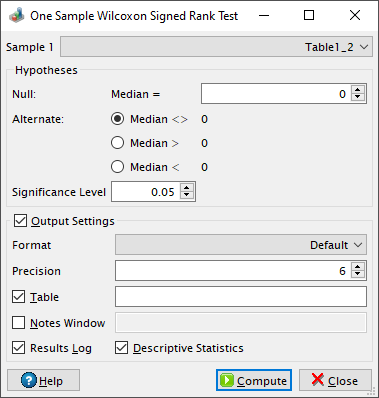# One Sample Wilcoxon Signed Rank Test Dialog

This dialog is activated by selecting the One Sample Wilcoxon Signed Rank Test... command from the Statistics -> Nonparametric Tests -> menu. It can be used as a non-parametric alternative to a one-sample t-test. The test determines whether the median of the data sample is equal to a specific value.

Figure 5-93. The one sample Wilcoxon signed rank test dialog.QtiPlot computes the test statistic, W, and a Z-score. If the reduced sample size, `Nr`, after excluding the ties, is more than 200, QtiPlot uses the normal distribution approximation in order to calculate a p-value. Bellow this threshold QtiPlot also uses an exact method to compute the statistic probabilities using a recursion equation explained by Jerome Klotz in his book from 2006, A Computational Approach to Statistics, chapter 15.2, pages 297-299. The null hypothesis is rejected if the probability is lower than the value of the significance level.

The Z-score is computed using the following formula: Z = [W-m-0.5*sign(W-m)]/[m(2Nr+1)/6]1/2, where 0.5 is a continuity correction factor and m = Nr(Nr+1)/4.